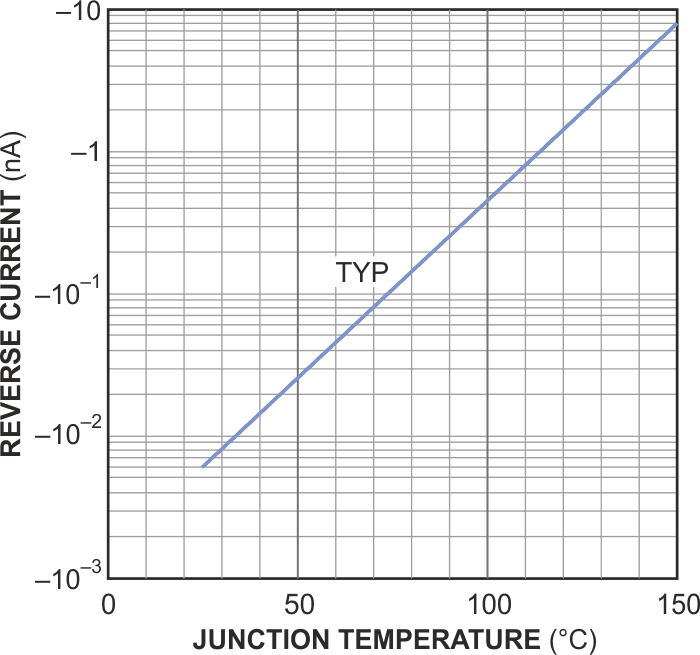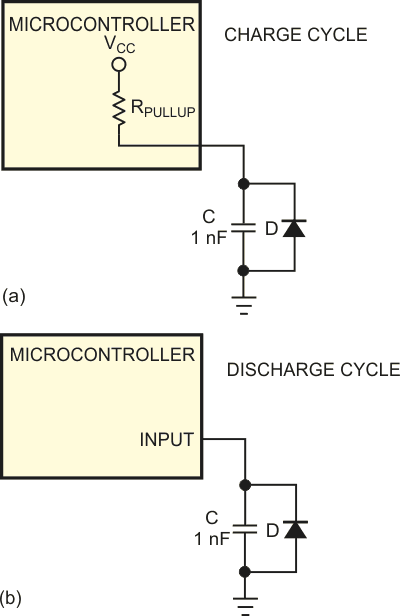Simple microcontroller-temperature measurement uses only a diode and a capacitor

Using a PN-junction diode for temperature measurement usually depends on its 2 mV/K temperature coefficient. Conventionally, you must amplify and digitize this voltage with an ADC before you can use the value in a microcontroller. Less well-known is the fact that the reverse current of a PN-junction diode shows a good exponential dependency over temperature; increasing the temperature by approximately 12 K doubles the leakage (Figure 1). An easy way to measure current over such a large range of two to three decades is to charge and discharge a capacitor and measure the time or frequency.Figure 1. The reverse current of a PN-junction diode shows an exponential dependency over temperature; increasing the temperature by approximately 12 K doubles the leakage.

A general-purpose I/O pin of a microcontroller charges a capacitor either by using it temporally as an output or by enabling a pull-up resistor, which is available in some controllers (Figure 2a). After charging the pin, you configure it as a high-impedance input, and a capacitor discharges through the leakage current of the diode (Figure 2b). The discharge time then is proportional to the temperature of the diode; thus, the diode exhibits exponential behavior. Depending on the type of diode, the exponential behavior can be nearly ideal. Calibration of a base point is necessary because the absolute value of the current varies greatly at a given temperature.Figure 2. Capacitor C first charges through the pull-up resistance of the microcontroller’s I/O pin configured as an output (a). The capacitor then discharges through the reverse leakage of diode D (b).

Selecting the diode and the value of the capacitor requires some care. The smaller the PN junction, the smaller the reverse current and the longer the discharging time. Periods longer than a few seconds are usually unsuitable. Making the capacitor’s value too low leads to errors because the capacitance of any cable and the capacitance of the PN-junction diode come into effect.

Typically, a power diode, such as a 1N4001 with a capacitance of 1 nF, gives suitable results. The discharge time is approximately 0.3 to 1 sec at room temperature, falling into the millisecond range at 100 °C. The PN-junction diode of a power transistor should also work.

EDN